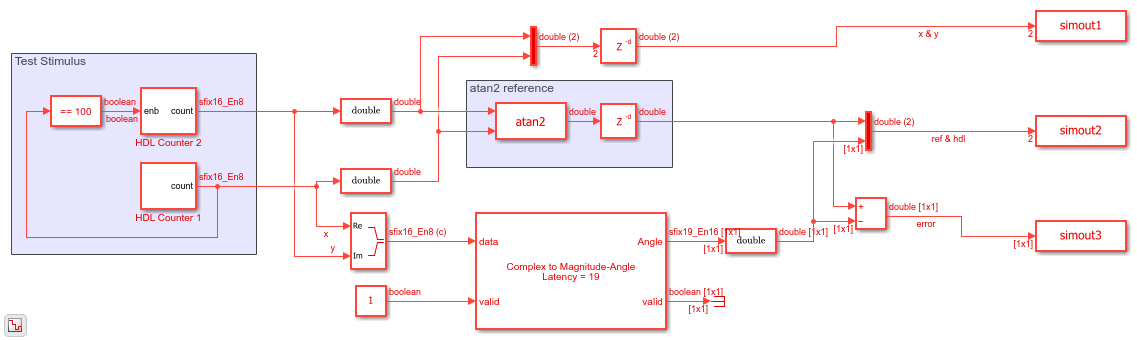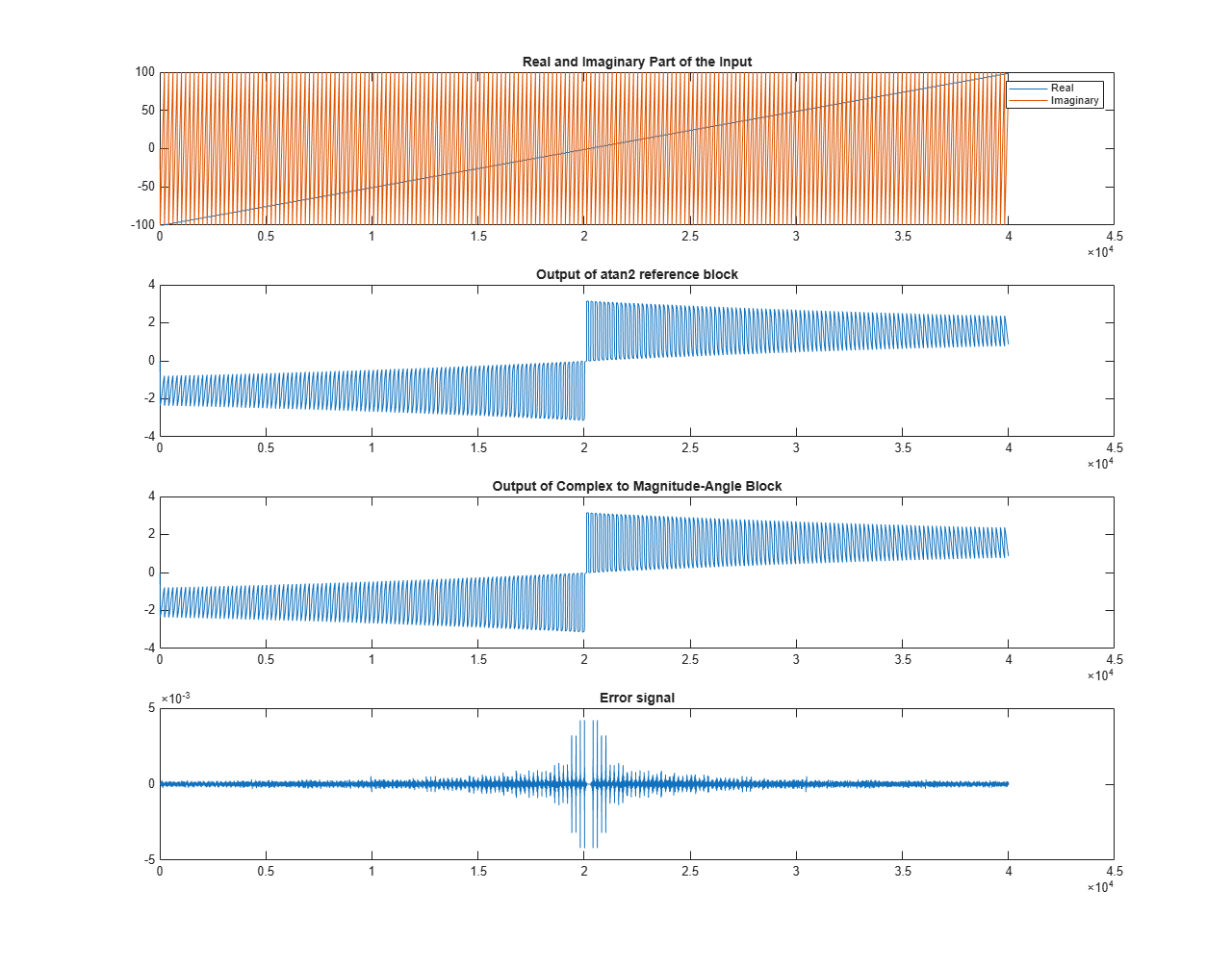# Implement `atan2` Function for HDL

This example shows how to use the Complex to Magnitude-Angle block to implement the `atan2` function in hardware.

This example model compares the output of Complex to Magnitude-Angle block with the `atan2` function implemented using Trigonometric Function block.

HDL Counter 1 and HDL Counter 2 blocks generate the real and imaginary parts, respectively, of the complex number.

Real-Imag to Complex block constructs the complex output from real and imaginary inputs.

Trigonometric Function block with function parameter set as `atan2` is used to generate the reference output angle. This block uses the CORDIC approximation to calculate the angle.

The Complex to Magnitude-Angle block is configured to return the angle in radians produces the angle of the complex input as an output. This block also models the latency of the hardware implementation.

To align the data for comparison, the reference data path includes a delay block with the same latency.

The Complex to Magnitude-Angle block supports HDL code generation, if you add it to a subsystem.

```modelname = 'HDLatan2Example'; open_system(modelname); set_param(modelname,'SampleTimeColors','on'); set_param(modelname,'SimulationCommand','Update'); set_param(modelname,'Open','on'); set(allchild(0),'Visible','off'); out = sim(modelname); ```Compare the outputs of Complex to Magnitude-Angle block against the atan2 function block.

```figure('units','normalized','outerposition',[0 0 1 1]) subplot(4,1,1) plot(simout1(:,1)) hold on; plot(simout1(:,2)) hold off; legend('Real','Imaginary') title('Real and Imaginary Part of the Input') subplot(4,1,2) plot(simout2(:,1)) title('Output of atan2 reference block') subplot(4,1,3) plot(simout2(:,2)) title('Output of Complex to Magnitude-Angle Block') subplot(4,1,4) plot(simout3) title('Error signal') ```